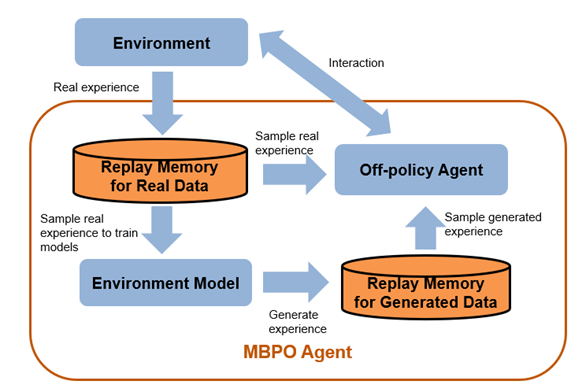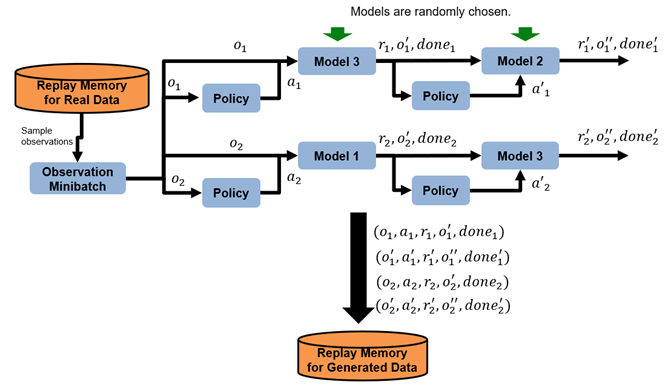## Model-Based Policy Optimization (MBPO) Agents

Model-based policy optimization (MBPO) is a model-based, online, off-policy reinforcement learning algorithm. For more information on the different types of reinforcement learning agents, see Reinforcement Learning Agents.

The following figure shows the components and behavior of an MBPO agent. The agent samples real experience data through environmental interaction and trains a model of the environment using this experience. Then, the agent updates the policy parameters of its base agent using the real experience data and experience generated from the environment model.Note

MBPO agents do not support recurrent networks.

MBPO agents can be trained in environments with the following observation and action spaces.

Observation SpaceAction Space
ContinuousDiscrete or continuous

You can use the following off-policy agents as the base agent in an MBPO agent.

Action SpaceBase Off-Policy Agent
Discrete
Continuous

MBPO agents use an environment model that you define using an `rlNeuralNetworkEnvironment` object, which contains the following components. In general, these components use a deep neural network to learn the environment behavior during training.

During training, an MBPO agent:

• Updates the environment model at the beginning of each episode by training the transition functions, reward function, and is-done function

• Generates samples using the trained environment model and stores the samples in a circular experience buffer

• Stores real samples from the interaction between the agent and the environment using a separate circular experience buffer within the base agent

• Updates the actor and critic of the base agent using a mini-batch of experiences randomly sampled from both the generated experience buffer and the real experience buffer

### Training Algorithm

MBPO agents use the following training algorithm, in which they periodically update the environment model and the base off-policy agent. To configure the training algorithm, specify options using an `rlMBPOAgentOptions` object.

1. Initialize the actor and critics of the base agent.

2. Initialize the transition functions, reward function, and is-done function in the environment model.

3. At the beginning of each training episode:

1. For each model-training epoch, perform the following steps. To specify the number of epochs, use the `NumEpochForTrainingModel` option.

1. Train the transition functions. If the corresponding `LearnRate` optimizer option is `0`, skip this step.

• Use a half-mean loss for an `rlContinuousDeterministicTransitionFunction` object and a maximum likelihood loss for an `rlContinuousStochasticTransitionFunction` object.

• To make each observation channel equally important, first compute the loss for each observation channel. Then, divide each loss by the number of elements in its corresponding observation specification.

`$Loss=\sum _{i=1}^{{N}_{o}}\frac{1}{{M}_{oi}}Los{s}_{oi}$`

For example, if the observation specification for the environment is defined by `[rlNumericSpec([10.1]) rlNumericSpec([4,1])]`, then No is 2, Mo1 is 10, and Mo2 is 4.

2. Train the reward function. If the corresponding `LearnRate` optimizer option is `0` or a ground-truth custom reward function is defined, skip this step.

• Use a half-mean loss for an `rlContinuousDeterministicRewardFunction` object and a maximum likelihood loss for an `rlContinuousStochasticRewardFunction` object.

3. Train the is-done function. If the corresponding `LearnRate` optimizer option is `0` or a ground-truth custom is-done function is defined, skip this step.

• Use a weighted cross-entropy loss function. In general, the terminal conditions (`isdone = 1`) occur much less frequently than nonterminal conditions (`isdone = 0`). To deal with the heavily imbalanced data, use the following weights and loss function.

`$\begin{array}{l}{w}_{0}=\frac{1}{{\sum }_{i=1}^{M}\left(1-{T}_{i}\right)},\text{ }{w}_{1}=\frac{1}{{\sum }_{i=1}^{M}{T}_{i}}\\ Loss=\frac{-1}{M}\sum _{i=1}^{M}\left({w}_{0}{T}_{i}\mathrm{ln}{Y}_{i}+{w}_{1}\left(1-{T}_{i}\right)\mathrm{ln}\left(1-{Y}_{i}\right)\right)\end{array}$`

Here, M is the mini-batch size, Ti is a target, and Yi is the output from the reward network for the ith sample in the batch. Ti = 1 when `isdone` is 1 and Ti = 0 when `isdone` is 0.

2. Generate samples using the trained environment model. The following figure shows an example of two roll-out trajectories with a horizon of two.1. Increase the horizon based on the horizon update settings defined in the `ModelRolloutOptions` object.

2. Randomly sample a batch of NR observations from the real experience buffer. To specify NR, use the `ModelRolloutOptions.NumRollout` option.

3. For each horizon step:

• Randomly divide the observations into NM groups, where NM is the number of transition models, and assign each group to a transition model.

• For each observation oi, generate an action ai using the exploration policy defined by the `ModelRolloutOptions.NoiseOptions` object. If `ModelRolloutOptions.NoiseOptions` is empty, use the exploration policy of the base agent.

• For each observation-action pair, predict the next observation o'2 using the corresponding transition model.

• Using the environment model reward function, predict the reward value ri based on the observation, action, and next observation.

• Using the environment model is-done function, predict the termination signal donei based on the observation, action, and next observation.

• Add the experience (oi,ai,ri,o'i,donei) to the generated experience buffer.

• For the next horizon step, substitute each observation with the predicted next observation.

4. For each step in each training episode:

1. Sample a mini-batch of M total experiences from the real experience buffer and the generated experience buffer. To specify M, use the `MiniBatchSize` option.

• Sample Nreal = ⌈M·R samples from the real experience buffer. To specify R, use the `RealRatio` option.

• Nmodel = MNreal samples from the generated experience buffer.

2. Train the base agent using the sampled mini-batch of data by following the update rule of the base agent. For more information, see the corresponding SAC, TD3, DDPG, or DQN training algorithm.

### Tips

• MBPO agents can be more sample-efficient than model-free agents because the model can generate large sets of diverse experiences. However, MBPO agents require much more computational time than model-free agents, because they must train the environment model and generate samples in addition to training the base agent.

• To overcome modeling uncertainty, best practice is to use multiple environment transition models.

• If they are available, it is best to use known ground-truth reward and is-done functions.

• It is better to generate a large number of trajectories (thousands or tens of thousands). Doing so generates many samples, which reduces the likelihood of selecting the same sample multiple times in a training episode.

• Since modeling errors can accumulate, it is better to use a shorter horizon when generating samples. A shorter horizon is usually enough to generate diverse experiences.

• In general, an agent created using `rlMBPOAgent` is not suitable for environments with image observations.

• When using a SAC base agent, taking more gradient steps (defined by the `NumGradientStepsPerUpdate` SAC agent option) makes the MBPO agent more sample-efficient. However, doing so increases the computational time.

• The MBPO implementation in `rlMBPOAgent` is based on the algorithm in the original MBPO paper  but with the differences shown in the following table.

Original Paper`rlMBPOAgent`
Generates samples at each environment stepGenerates samples at the beginning of each training episode
Trains actor and critic using only generated samplesTrains actor and critic using both real data and generated data
Uses stochastic environment modelsUses either stochastic or deterministic environment models
Uses SAC agentsCan use SAC, DQN, DDPG, and TD3 agents

 Janner, Michael, Justin Fu, Marvin Zhang, and Sergey Levine. “When to Trust Your Model: Model-Based Policy Optimization.” In Proceedings of the 33rd International Conference on Neural Information Processing Systems, 12519–30. 1122. Red Hook, NY, USA: Curran Associates Inc., 2019.